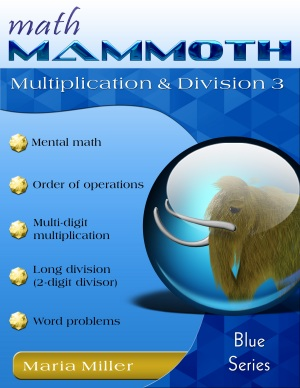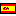^
You are here: HomeBlue Series → Multiplication Division 3

Math Mammoth Multiplication Division 3115 pages

Prices & ordering

Printed copy \$16.55

(credit cards, Paypal, PO)Este libro en español

Math Mammoth Multiplication Division 3 covers multiplication and division related topics, such as the multiplication algorithm, long division, estimation, divisibility, factoring, and ratios. This book also contains many lessons that aim to develop students' algebraic thinking, and get them used to simple equations. The book is aimed for 5th grade but can probably easily be used in 6th grade as well.

The PDF version of this book is enabled for annotation. This means that if you prefer, the student can fill it in on the computer, using the typewriter and drawing tools in Adobe Reader version 9 or greater.

One important line of thought throughout this book is that of equations. Students encounter the exact definition of an equation and an expression. They practice the order of operations with problems that also reinforce the idea of the equal sign ("=") as denoting equality of the right and left sides of an equation. These kind of exercises are needed because children may think that an equal sign signifies the act of finding the answer to a problem (as in 134 + 23 = ?, for example), which is not so.

Simple diagrams are used to illustrate simple multiplication and division equations and mixture equations, such as 4x + 38 = 128. The idea is see how the four operations are used together in solving problems and in simple equations. We are trying to develop students' algebraic thinking, including the abilities to: translate problems into mathematical operations, comprehend the many operations needed to yield an answer to a problem, "undo" operations, and so on. Many of the ideas here are preparing them for algebra in advance.

In the middle of the book are lessons on multi-digit multiplication (multiplying in columns). These lessons go farther than just reviewing the well-known algorithm. We study in detail: multiplying in parts (partial products), how those partial products can be seen in the algorithm itself, and how multi-digit multiplication can be visualized geometrically.

Students also practice long division, including two-digit divisors. Then we study divisibility, prime numbers, and factoring, including the sieve of Eratosthenes.

The lesson Introduction to Ratios is important, as it shows the connection between fractional parts, ratios, and bar models.

You can make additional worksheets for long multiplication and long division on this web page. Scroll down to the multiplication and division sections.

You might also be interested in:
• Math Mammoth Division 2—a worktext about long division, word problems, average, and divisibility (grades 4-5).
• Math Mammoth Multiplication 2—a worktext about multi-digit multiplication, order of operations, estimation, and word problems (grade 4).
• Make It Real Learning: Arithmetic I—an activity workbook with real-world data, focusing on situations that use addition, subtraction, estimation, division, bar graphs, and place value with large numbers (grades 3-6).

Better Yet - Bundle Deals!

*BONUS*: Buy any bundle listed above, and get the fully upgraded Soft-Pak software for FREE (includes 4 math and 2 language arts programs, complete with on screen and printable options).

How and where to order

You can buy Math Mammoth books at:

• Here at MathMammoth.com website — simply use the "Add to cart" buttons you see on the product pages.
• Rainbow Resource carries printed copies for the Light Blue series books, plus several CDs (Light Blue and Blue series).
• Homeschool Buyers Co-op offers download versions of the Light Blue series, plus the Blue series bundle.
• Lulu offers printed copies for the Blue, Golden, and Light Blue series books.

By purchasing any of the books, permission IS granted for the teacher (or parent) to reproduce this material to be used with his/her students in a teaching situation; not for commercial resale. However, you are not permitted to share the material with another teacher.

In other words, you are permitted to make copies for the students/children you are teaching, but not for other teachers' usage.

Math Mammoth books are PDF files. You will need Adobe Reader to view them, including if you use a Mac or Linux. You can try other PDF viewers, but they seem to either omit or mess up some of the images.
WAIT!

Receive my monthly collection of math tips & resources directly in your inbox — and get a FREE Math Mammoth book!You can unsubscribe at any time.

Math Mammoth Tour

Confused about the different options? Take a virtual email tour around Math Mammoth! You'll receive:

An initial email to download your GIFT of over 400 free worksheets and sample pages from my books. Six other "TOURSTOP" emails that explain the important things and commonly asked questions concerning Math Mammoth curriculum. (Find out the differences between all these different-colored series!)

This way, you'll have time to digest the information over one or two weeks, plus an opportunity to ask me personally about the curriculum.
A monthly collection of math teaching tips & Math Mammoth updates (unsubscribe any time)"Mini" Math Teaching Course

This is a little "virtual" 2-week course, where you will receive emails on important topics on teaching math, including:

- How to help a student who is behind
- Troubles with word problems
- Teaching multiplication tables
- Why fractions are so difficult
- The value of mistakes
- Should you use timed tests
- And more!

A GIFT of over 400 free worksheets and sample pages from my books right in the very beginning.A monthly collection of math teaching tips & Math Mammoth updates (unsubscribe any time)
Enter your email to receive math teaching tips, resources, Math Mammoth news & sales, humor, and more! I tend to send out these tips about once monthly, near the beginning of the month, but occasionally you may hear from me twice per month (and sometimes less often).• A GIFT of over 400 free worksheets and sample pages from my books.